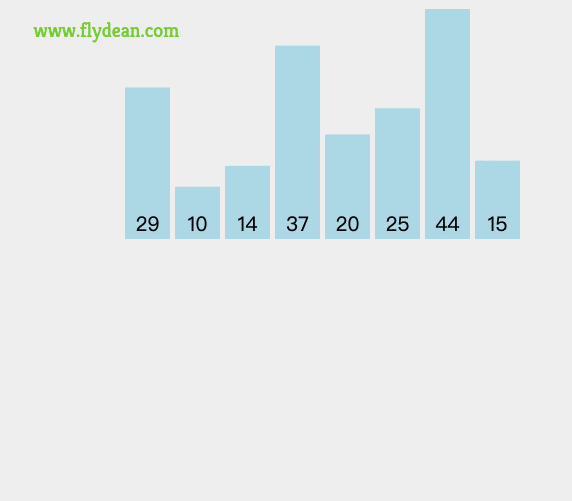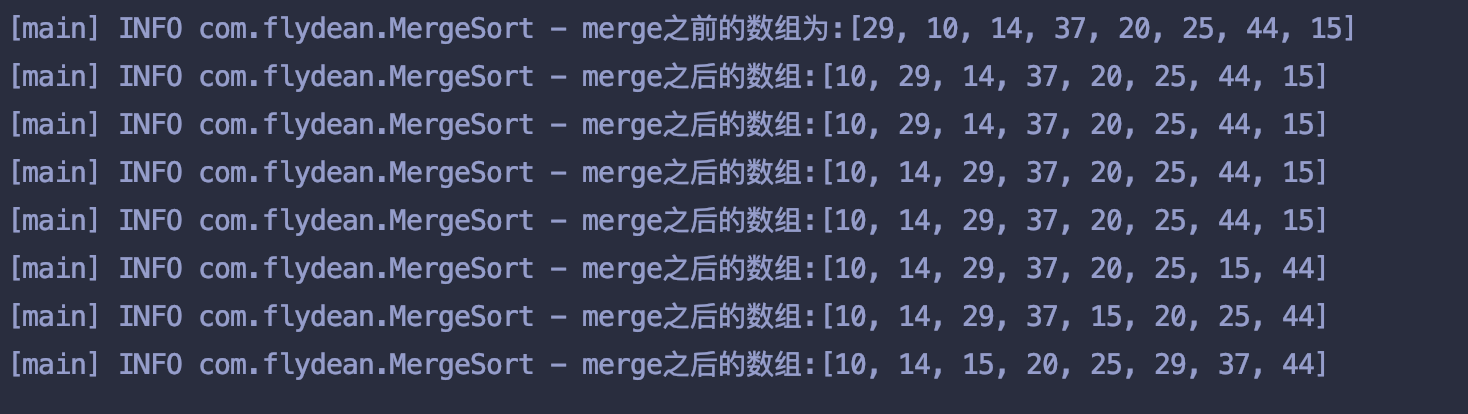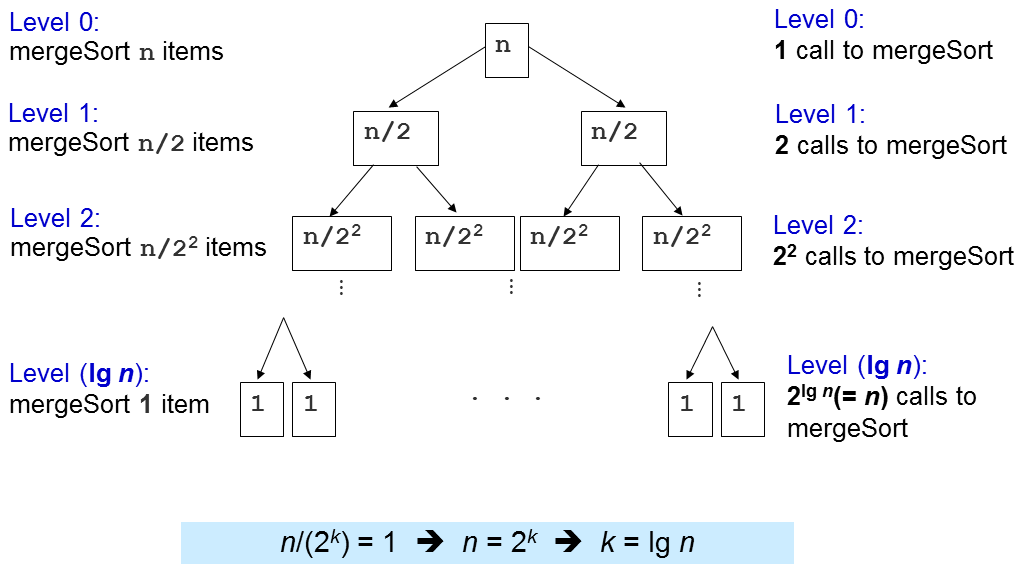# 归并排序的例子[29,10,14,37]又分成两部分[29,10]和[14,37]。

[29,10]又被分成两部分和，然后对和进行归并排序生成[10,29]。

# 归并排序的java实现

   /**
*合并两部分已排序好的数组
* @param array 待合并的数组
* @param low   数组第一部分的起点
* @param mid   数组第一部分的终点，也是第二部分的起点-1
* @param high  数组第二部分的终点
*/
private void  merge(int[] array, int low, int mid, int high) {
// 要排序的数组长度
int length = high-low+1;
// 我们需要一个额外的数组存储排序过后的结果
int[] temp= new int[length];
//分成左右两个数组
int left = low, right = mid+1, tempIdx = 0;
//合并数组
while (left <= mid && right <= high) {
temp[tempIdx++] = (array[left] <= array[right]) ? array[left++] : array[right++];
}
//一个数组合并完了，剩下的一个继续合并
while (left <= mid) temp[tempIdx++] = array[left++];
while (right <= high) temp[tempIdx++] = array[right++];
//将排序过后的数组拷贝回原数组
for (int k = 0; k < length; k++) array[low+k] = temp[k];
}


merge的逻辑就是对这两个数组进行合并。

    public void doMergeSort(int[] array, int low, int high){
// 要排序的数组 array[low..high]
//使用二分法进行递归，当low的值大于或者等于high的值的时候，就停止递归
if (low < high) {
//获取中间值的index
int mid = (low+high) / 2;
//递归前面一半
doMergeSort(array, low  , mid );
//递归后面一半
doMergeSort(array, mid+1, high);
//递归完毕，将排序过后的数组的两部分合并
merge(array, low, mid, high);
log.info("merge之后的数组:{}",array);
}
}


array是原数组，low和high标记出了要递归排序的数组起始位置。# 归并排序的时间复杂度divide方法将排序分成了logN层，每层都可以看做是对N个元素的合并排序，因此每层的时间复杂度是O(N)。

learn-algorithm